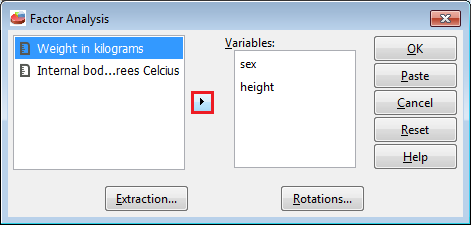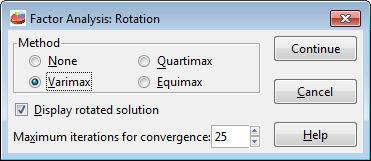# Section 6: Multivariate Analysis

A multivariate analysis is based on the statistical principle of multivariate statistics, which involves observation and analysis of more than one statistical outcome variable at a time.

## Performing a Factor Analysis

To conduct a factor analysis, click the "Analyze" drop-down menu then choose the Factor Analysis option as shown in figure 4-11 below.Figure 6-1

From the factor analysis window, choose each and every variable you would like to include in the data set then simply choose ok to return results in the output window.Figure 6-2

This is the extraction window. It grants numerous options such as to change the matrix type, display type and the maxium iterations for convergences.Figure 6-3

This window allows you to adjust the axis rotation. Make sure whichever method you choose, the Display rotated solution box is checked so it displays properly in the output window.Figure 6-4

This is the factor analysis output window.Figure 6-5